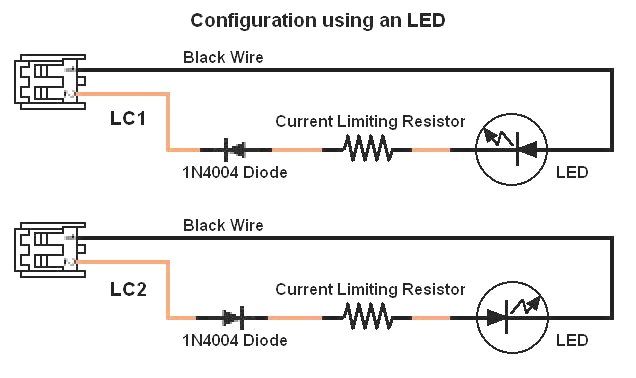To use LED's with the Mini Commander LC1 and LC2 outputs, you will need a series resistor and diode connected to the LED.  The Mini-Commander outputs 1/2 of the supply voltage on LC1 and LC2.  Each output has a different polarity, thus choose the circuit above depending on what output the LED is attached to. The series resistor is calculated as follows: Example 1: Source Voltage = 9 volts  Voltage Drop = 3.6 volts (Typical for a blue or white LED)  Desired Current = 20 milliamps (a typical value for White)  To calculate the resistor you need: (9 - 3.6) / ( 20 / 1000 ) = 270 ohms Example 2: Source Voltage = 9 volts  Voltage Drop = 1.4 volts (Typical for a Red LED)  Desired Current = 15 milliamps (a typical value for Red)  To the calculate the resistor you need: (9 - 1.4) / ( 15 / 1000 ) = 510 ohms